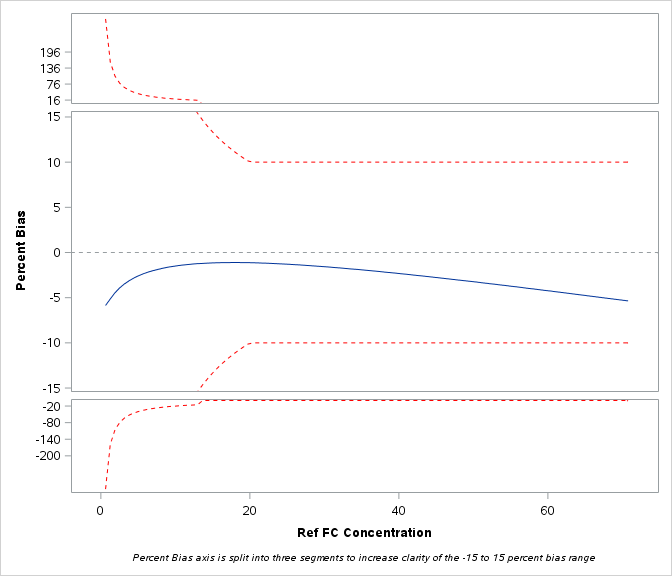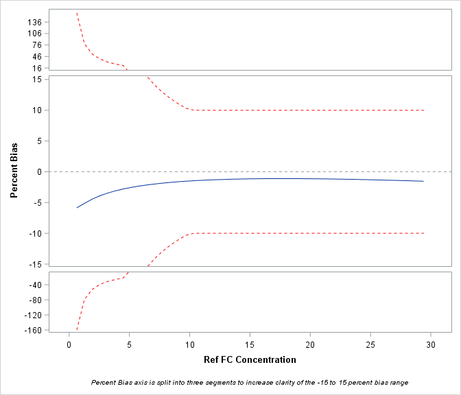## sgplot template

Hi, when I tried to generate a sgplot using the macro below, here I separated the plot to 3 different areas.  When the lower band (lower) locate in both middle and bottom areas (across the -15 on y axis), on the top of area3 (<-15) also showed a red line as pasted below even if the real Y values are not located in that area. Is any way to correct this display to show the red line plot as it is?

Thank you!

Limin Shu

%macro TextBias;

goptions reset=all;

ods graphics/ imagemap=on width=7in Height=6in ;
proc template;
define statgraph sgplot1;
begingraph;
%if &biasarea=3 %then %do;
layout lattice / rows=3 rowweights=(.2 .6 .2) rowdatarange=union rowgutter=2 columndatarange=unionall;
%end;
%else %do;
layout lattice / rows=1 rowweights=(1) rowdatarange=union rowgutter=2 columndatarange=unionall;
%end;
%if &sumbias2 ge 1 or &sumlimit2 ge 1 %then %do;
layout overlay /xaxisopts=(display=none )
yaxisopts=(display=(tickvalues ticks)
linearopts=(viewmin=16 tickvaluesequence=(start=16 end=200 increment=10)));
%if &sumbias2 ge 1 %then %do;
seriesplot X=FC_Dose Y=Percent_bias2;
%end;
%if &sumlimit2 ge 1 %then %do;
seriesplot X=FC_Dose Y=Upper2/lineattrs=(color=red pattern=2);
%end;
endlayout;
%end;

%if &sumbias1 ge 1 or &sumlimit1 ge 1 %then %do;
layout overlay /xaxisopts=(display=none)
yaxisopts=(label="Percent Bias" labelattrs=(weight=bold) linearopts=(viewmin=-15 viewmax=15 tickvaluesequence=(start=-15 end=15 increment=5)));
Referenceline y=0/lineattrs=(pattern=2);

%if &sumbias1 ge 1 %then %do;
seriesplot X=FC_Dose Y=Percent_bias;
%end;
%if &sumlimit1 ge 1 %then %do;
seriesplot X=FC_Dose Y=lower/lineattrs=(color=red pattern=2);
seriesplot X=FC_Dose Y=Upper/lineattrs=(color=red pattern=2);
%end;
endlayout;
%end;

%if &sumbias3 ge 1 or &sumlimit3 ge 1 %then %do;
layout overlay /xaxisopts=(display=none)
yaxisopts=(display=(tickvalues ticks)
linearopts=(viewmax=-16 tickvaluesequence=(start=-200 end=-16 increment=10)));
%if &sumbias3 ge 1 %then %do;
seriesplot X=FC_Dose Y=Percent_bias3;
%end;

%if &sumlimit3 ge 1 %then %do;
seriesplot X=FC_Dose Y=Lower3/lineattrs=(color=red pattern=2);
%end;

endlayout;
%end;

columnaxes;
columnaxis / offsetmin=.05 offsetmax=.05 display=(label tickvalues ticks) discreteopts=(tickvaluefitpolicy=thin ) label="Ref FC Concentration"

labelattrs=(weight=bold);
endcolumnaxes;
%if &sumbias2 ge 1 or &sumlimit2 ge 1 %then %do;
entryfootnote "Percent Bias axis is split into three segments to increase clarity of the -15 to 15 percent bias range"/ textattrs=(size=7pt style=italic);
%end;
endlayout;
endgraph;
end;
run;

proc sort data=save.Reeses2b;
by FC_Dose;
run;
proc sgrender data= save.Reeses2b template=sgplot1;
run;

%mend TextBias;6 REPLIES 6

## Re: sgplot template

That's next to impossible to debug.  First off, can't see any data.

As a suggestion I would read up on proc template and dynamic variables. The macro here is not needed an obfuscates the code a lot, for instance;

```goptions reset=all;

ods graphics/ imagemap=on width=7in Height=6in ;

proc template;
define statgraph sgplot1;
begingraph;

dynamic biasarea biaw;

layout lattice / rows=biasarea rowweights=(biaw) rowdatarange=union rowgutter=2 columndatarange=unionall;
...

run;

proc sgrender...;
dynamic biasarea=3 biaw=".2 .6 .2";
run; ```

Note also the use of the code window (its the {i} above post area) which retains formatting and such like and makes code easier to read.

## Re: sgplot template

Thank you very much for your quick reply and apologize the code is not listed as formatted. The issue is when the area selected to be 3, the bottom area would display the curve that would be not expected. If I follow your dynamic setting, probably I still could not resolve this issue?

Limin

## Re: sgplot template

Hi Draycut,

Thank you. I just created a data as attached. And I also used my lengthy code to run it, surprisingly, I got the plot as expected. However, the same data in the enterprise guild would give the plot. So it must be the running environment cause the display difference?

Thank you!

The code in local 9.4 sas:

PROC IMPORT OUT= WORK.test
DATAFILE= "D:\Data\FC 2Pt B
ias Data.xlsx"
DBMS=EXCEL REPLACE;
RANGE="Sheet1\$";
GETNAMES=YES;
MIXED=NO;
SCANTEXT=YES;
USEDATE=YES;
SCANTIME=YES;
RUN;

%global sumbias1 sumbias2 sumbias3 sumlimit1 sumlimit2 sumlimit3 biasarea;

%macro TextBias;

goptions reset=all;

proc sort data=test;
by FC_Dose;
run;

Data test;
set test end=eof;
retain sumbias1 sumbias2 sumbias3 sumlimit1 sumlimit2 sumlimit3;
if Percent_bias > 20 then do; percent_bias2 = percent_bias; bias2 = 1;end; else do;bias2 = 0; percent_bias2=0; end;
sumbias2+bias2;
if upper > 20 then do; upper2=upper; limit2 = 1; end; else do; upper2=0; limit2 = 0;end;
sumlimit2+limit2;
if Percent_bias < -20 then do; percent_bias3 = percent_bias; bias3 = 1; end; else do;bias3 = 0; percent_bias3=0; end;
sumbias3+bias3;
if lower < -20 then do; lower3 =lower; limit3 = 1; end; else do; lower3 =0; limit3 = 0;end;
sumlimit3+limit3;
if -20 le Percent_bias le 20 then do; bias1=1;end; else do;bias1 = 0; end;
sumbias1+bias1;
if 0 le upper le 20 then do; limit1 = 1;end; else do;limit1 = 0; end;
sumlimit1+limit1;

if eof then do;
if sumbias1 ge 1 or sumlimit1 ge 1 then biasarea1=1; else biasarea1=0;
if sumbias2 ge 1 or sumlimit2 ge 1 then biasarea2=1; else biasarea2=0;
if sumbias3 ge 1 or sumlimit3 ge 1 then biasarea3=1; else biasarea3=0;
biasarea = biasarea1+biasarea2+biasarea3;
call symput('sumbias1', sumbias1);
call symput('sumbias2', sumbias2);
call symput('sumbias3', sumbias3);
call symput('sumlimit1', sumlimit1);
call symput('sumlimit2', sumlimit2);
call symput('sumlimit3', sumlimit3);
call symput('biasarea', biasarea);
end;
run;

ods graphics/ imagemap=on width=7in Height=6in ;
proc template;
define statgraph sgplot1;
begingraph;
%if &biasarea=3 %then %do;
layout lattice / rows=3 rowweights=(.2 .6 .2) rowdatarange=union rowgutter=2 columndatarange=unionall;
%end;
%else %do;
layout lattice / rows=1 rowweights=(1) rowdatarange=union rowgutter=2 columndatarange=unionall;
%end;
%if &sumbias2 ge 1 or &sumlimit2 ge 1 %then %do;
layout overlay /xaxisopts=(display=none )
yaxisopts=(display=(tickvalues ticks)
linearopts=(viewmin=16 tickvaluesequence=(start=16 end=200 increment=10)));
%if &sumbias2 ge 1 %then %do;
seriesplot X=FC_Dose Y=Percent_bias2;
%end;
%if &sumlimit2 ge 1 %then %do;
seriesplot X=FC_Dose Y=Upper2/lineattrs=(color=red pattern=2);
%end;
endlayout;
%end;
%if &sumbias1 ge 1 or &sumlimit1 ge 1 %then %do;
layout overlay /xaxisopts=(display=none)
yaxisopts=(label="Percent Bias" labelattrs=(weight=bold) linearopts=(viewmin=-15 viewmax=15 tickvaluesequence=(start=-15 end=15 increment=5)));
Referenceline y=0/lineattrs=(pattern=2);
%if &sumbias1 ge 1 %then %do;
seriesplot X=FC_Dose Y=Percent_bias;
%end;
%if &sumlimit1 ge 1 %then %do;
seriesplot X=FC_Dose Y=lower/lineattrs=(color=red pattern=2);
seriesplot X=FC_Dose Y=Upper/lineattrs=(color=red pattern=2);
%end;
endlayout;
%end;
%if &sumbias3 ge 1 or &sumlimit3 ge 1 %then %do;
layout overlay /xaxisopts=(display=none)
yaxisopts=(display=(tickvalues ticks)
linearopts=(viewmax=-16 tickvaluesequence=(start=-200 end=-16 increment=10)));
%if &sumbias3 ge 1 %then %do;
seriesplot X=FC_Dose Y=Percent_bias3;
%end;
%if &sumlimit3 ge 1 %then %do;
seriesplot X=FC_Dose Y=Lower3/lineattrs=(color=red pattern=2);
%end;
endlayout;
%end;
columnaxes;
columnaxis / offsetmin=.05 offsetmax=.05 display=(label tickvalues ticks) discreteopts=(tickvaluefitpolicy=thin ) label="Ref FC Concentration"
labelattrs=(weight=bold);
endcolumnaxes;
%if &sumbias2 ge 1 or &sumlimit2 ge 1 %then %do;
entryfootnote "Percent Bias axis is split into three segments to increase clarity of the -15 to 15 percent bias range"/ textattrs=(size=7pt style=italic);
%end;
endlayout;
endgraph;
end;
run;
proc sort data=test;
by FC_Dose;
run;
proc sgrender data= test template=sgplot1;
run;

%mend TextBias;

%TextBias;When running in the enterprise guide previously the disply looks (the bottom red line is not as expected):## Re: sgplot template

Hi draycut,

Please ignore my previous post. It may be not worthy to look at this long code and compare between the sas pc and enterprise guide. So please do not spend time on this and ignore this posted issue.

Thanks again!

Limin

## Re: sgplot template

As noted in my previous post, there are some things to do with your code which will make your life a lot easier, and people who have to look at you code:

First don't code all in mixed case - it sounds like shouting and makes the code hard to read.

Use indentations to show where blocks start/end.

In the datastep, you can use a select () clause to simplify that datastep

Thirdly, use macro variables only when needed.  In your example they are not needed, refer to the code I posted with dynamic variables, this again will halve the size of your proc template code by removing all the macro nonsense.

Discussion stats
• 6 replies
• 1274 views
• 0 likes
• 3 in conversation